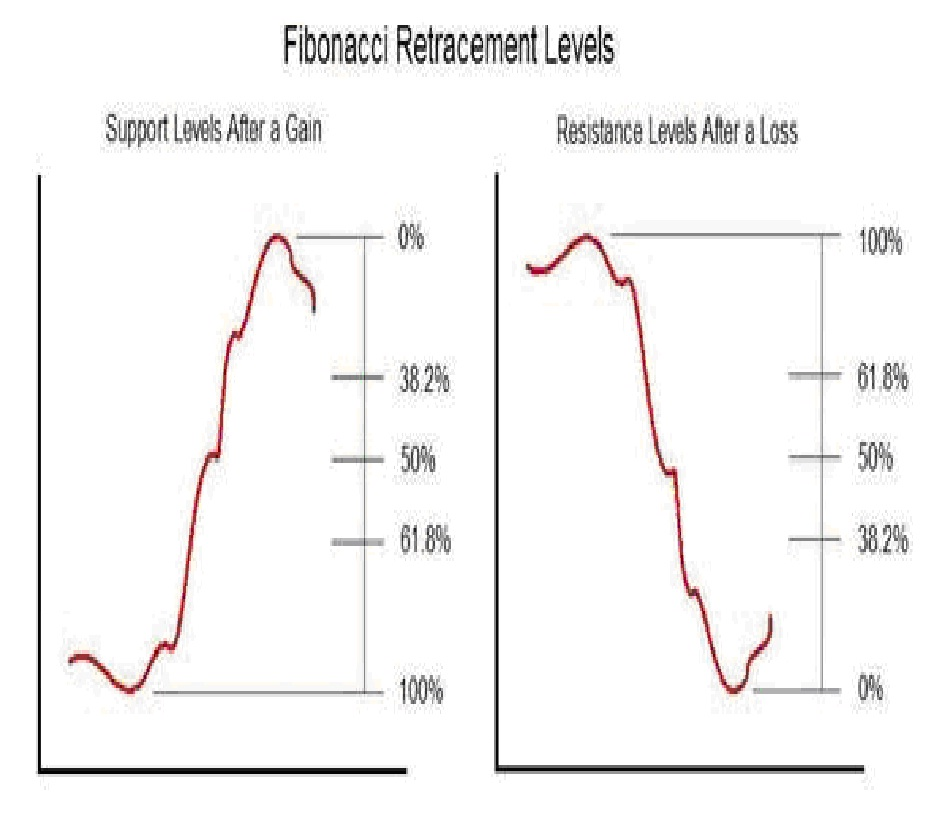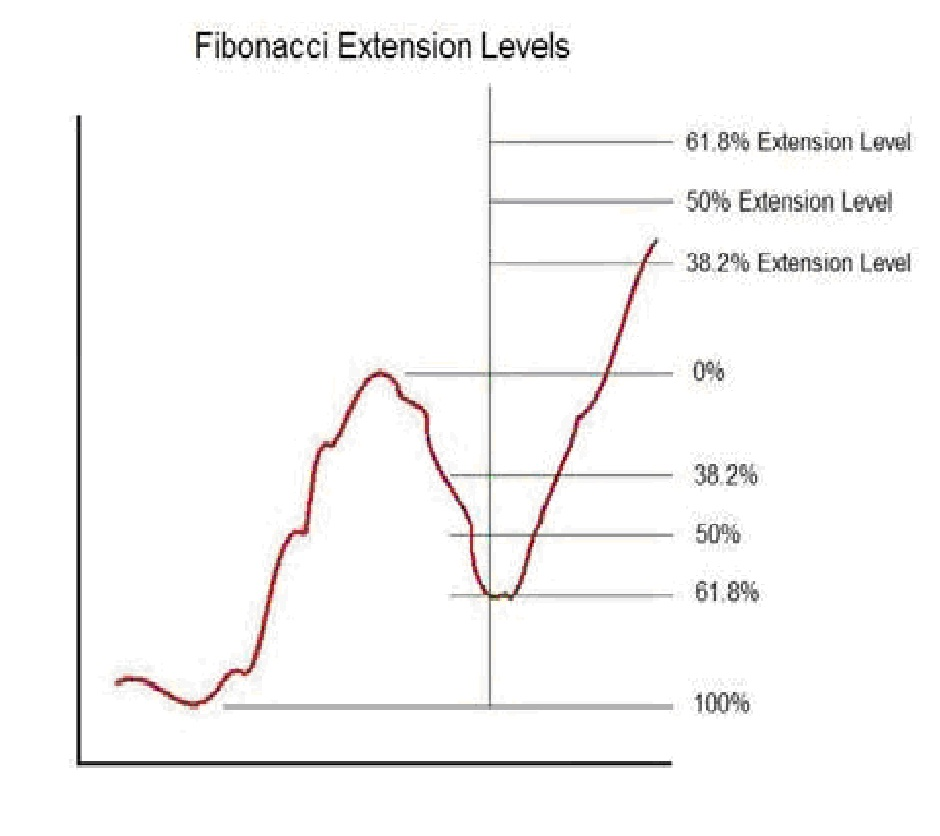Fibonacci explained- By Mark Deaton

Fibonacci explained - the following is a breakdown of how you can use Fibonacci in your stock, options and forex trading.
The Fibonacci sequence is a mathematical sequence in which each number, after two starting values, is the sum of the two preceding numbers. The sequence runs:
0, 1, 1, 2, 3, 5, 8, 13, 21, 34, 55, 89, 144, 233, 377, 610, 987, 1597â€¦â€¦â€¦

Dividing a Fibonacci number by its immediate predecessor yields an approximation of the Golden Ratio (roughly 1.6180327868852). The higher the Fibonacci number, the closer the approximation to the Golden Ratio becomes.

The sequence is named for medieval mathematician Leonardo of Pisa, commonly known as Fibonacci, who was responsible for introducing the Hindu-Arabic numeral system we currently use to Europe. Prior to Fibonacci, Roman numerals held sway.

Fibonacciâ€™s namesake sequence stems from his solution to the problem of modeling the growth of rabbit populations under ideal conditions, although it has since been used in many other contexts.

Today Fibonacci numbers are used extensively in the study of mathematics, computer science and biology. The Golden Ratio shows up music, art and architecture and is often used as a guide for creating visually pleasing proportions.

Perhaps because it is relatively easy to understand, the Fibonacci sequence is also frequently referenced in pop culture. For example, it makes a prominent appearance in The DaVinci Code as the password that opens the codex.

In finance, Fibonacci numbers, or more precisely the Golden Ratio, form the basis of a popular method of technical analysis. The idea is that in the aftermath of a significant price movement, subsequent levels of support and resistance will form around â€˜Fibonacci Significantâ€™ numbers, usually 38.2%, 50% and 61.8%, which are percentages of the total price drop or gain.After a large price movement, technical traders pay particular attention to these retracement levels. For example, if the price of a stock has recently shot up, a technical trader might sell until the price declined to the 38.2% level and begin buying when it reached the 61.8% level.
Conversely, after a big decline, the same trader might buy until the price recovered to the 38.2% level and begin selling as the price approached the 61.8% level.

Fibonacci Extensions are, appropriately enough, an extension of the same idea. Technical traders have observed that after a period of retracement, stock prices often resume moving in accordance with their original trend.
Extending the Fibonacci analysis allows technical traders to predict the next turning point. Fibonacci Extension levels are calculated based on the original price movement.Having observed a major price increase in a stock, a technical trader might begin buying when the price had subsequently declined to the 61.8% level, then hold the stock until the price approached the 61.8% extension level, at which point it would be time to sell in anticipation of a new retracement. Extensions are applied in a similar manner for price declines, with the expectation being that after a retracement, the price decline will resume.

Fibonacci Effectiveness
There is not any strictly rational reason why stock prices should behave as Fibonacci analysis predicts. While it is true that the Golden Ratio appears frequently in nature, this does not in any way imply that we should expect it to play a role in financial markets. After all, rabbit population growth has very little to do with stock prices.

However, it would be a mistake to dismiss Fibonacci methods as useless superstition. The fact is that there are many active share traders who use Fibonacci retracements and extensions to guide their trading strategy.
If enough traders use and act on Fibonacci analysis, the method will work, regardless of whether or not it has any rational basis. In the short term at least, even ill founded theories can move markets. Regardless of whether or not Fibonacci naturally influences the market, the use of this analysis by many traders leads to an overall self-fulfilling prophecy in stock prices.

Phenomena like this are not uncommon in markets, and in fact, market psychology is a major focus of study in the field of Behavioral Economics.
In essence, while Fibonacci retracements and extensions may not have any real basis from a strict financial analysis perspective, they are a useful tool for predicting the behavior of many traders operating in the market.
For this reason, Fibonacci analysis can be an effective part of an overall trading strategy.

The key is to develop an understanding of how other traders are applying Fibonacci analysis. Target selection is also important. If past price movements of a stock appear to conform to Fibonacci predictions, then it is likely that traders using Fibonacci analysis are active in the trading of that particular stock.

This in turn improves the odds that Fibonacci analysis will be effective in predicting the future movements of that stock. Applied with a thorough understanding of how and where other traders are using it, Fibonacci retracements and extensions can be solid enhancer of trading profits.

Author
Mark Deaton
www.Fibonaccigenius.com

One thought on “Fibonacci explained- By Mark Deaton”

1.Jacqueline on said:

Cool blog!